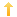## PowerBuilder – Determining if Controls Overlap

Posted on Wednesday, May 2nd, 2012 at 5:32 pm in

I had a situation where I needed to know if the user is clicking on a control which is underneath a rectangle on a datawindow. In this case the interior of the rectangle was transparent.

```ls_name = dwo.name

IF (left(ls_name, 2) = 'r_') THEN // my standard rectangle naming convention
ls_objects = this.Describe( 'DataWindow.Objects')
ls_objects = ls_objects + '~t'
ll_pos = pos(ls_objects, '~t')
ll_orig_pos = 1
ll_ypos = Pixelstounits(ypos, YPixelsToUnits!)
ll_xpos = Pixelstounits(xpos, XPixelsToUnits!)
// loop through objects on datawindow
DO WHILE ll_pos > 0
ls_object = mid(ls_objects, ll_orig_pos, ll_pos -ll_orig_pos)
// check if pointer is over something behind the rectangle
ls_x = this.Describe(ls_object + '.X')
ls_y = this.Describe(ls_object + '.Y')
ls_h = this.Describe(ls_object + '.height')
ls_w = this.Describe(ls_object + '.width')

IF NOT ((ll_xpos + 1) <= integer(ls_x)) AND NOT &
(ll_xpos >= (integer(ls_x) + integer(ls_w))) AND NOT &
((ll_ypos + 1) <= integer(ls_y)) AND NOT &
(ll_ypos >= (integer(ls_y) + integer(ls_h))) THEN
// cursor position is within boundaries of underlying control
is_site_location = ls_object
EXIT
END IF
ll_orig_pos = ll_pos + 1
ll_pos = pos(ls_objects, '~t', ll_orig_pos)
LOOP
END IF```

The following link to how to determine if rectangles overlap is here.

You might also be interested in
Tags: